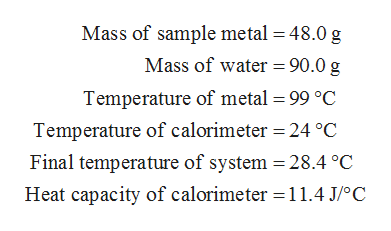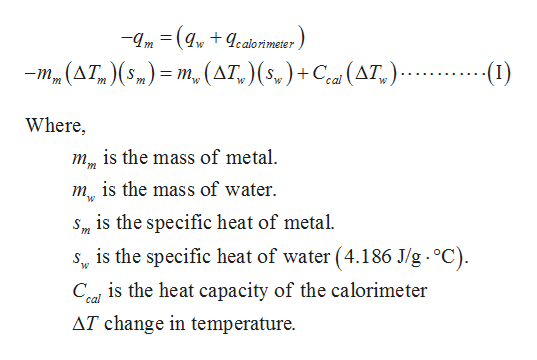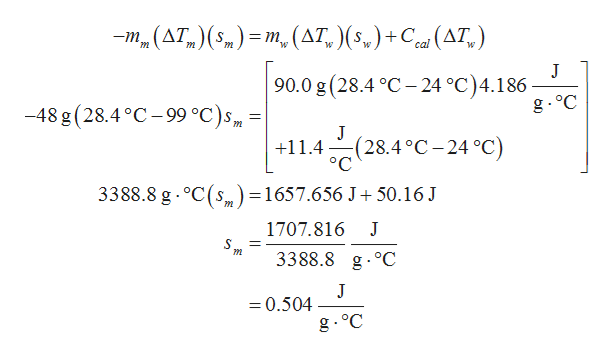# A 48.0−g sample of an unknown metal at 99°C was placed in a constant-pressure calorimeter containing 90.0 g of water at 24.0°C. The final temperature of the system was found to be 28.4°C. Calculate the specific heat of the metal. (The heat capacity of the calorimeter is 11.4 J/°C.)

Question

A 48.0−g sample of an unknown metal at 99°C was placed in a constant-pressure calorimeter containing 90.0 g of water at 24.0°C. The final temperature of the system was found to be 28.4°C. Calculate the specific heat of the metal. (The heat capacity of the calorimeter is 11.4 J/°C.)

check_circleExpert Solution
Step 1

Given data:help_outlineImage TranscriptioncloseMass of sample metal 48.0 g Mass of water = 90.0 g Temperature of metal 99 °C Temperature of calorimeter 24 °C Final temperature of system = 28.4 °C Heat capacity of calorimeter 11.4 J/oC fullscreen
Step 2

Heat lost by metal=heat gain by water and calorimeter.

Therefore,help_outlineImage Transcriptionclose-m( ealorimeter m_(AT ()= m(AT,)(s,)+ Ce(AT,). () w Where is the mass of metal mis the mass of water S m is the specific heat of metal. is the specific heat of water (4.186 J/g. °C) Ceal is the heat capacity of the calorimeter AT change in temperature. fullscreen
Step 3

Substitute all the given values in ...help_outlineImage Transcriptionclose-m (AT,) ( 5) = m. (AT, ) (5,) +C (AT) W J 90.0 g (28.4 C -24 °C)4.186 g oC -48 g (28.4 °C-99 °C)s J :(28.4°C-24 °C) ° C +11.4 3388.8 g oC(s=1657.656 J 50.16 J m 1707.816 S m 3388.8 g.°C J =0.504 g. °C fullscreen

### Want to see the full answer?

See Solution

#### Want to see this answer and more?

Solutions are written by subject experts who are available 24/7. Questions are typically answered within 1 hour*

See Solution
*Response times may vary by subject and question
Tagged in

### Chemistry